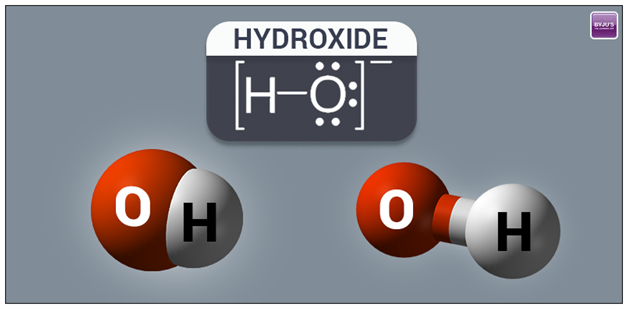# HydroxideHydroxide is a diatomic anion consisting of one atom of oxygen and hydrogen bonded together by a covalent bond, with chemical formula as OH- and carries negatively charged ion. It functions as a nucleophile, a ligand, a catalyst, and a base.

Ion of a metal is usually the positively charged part of the compound, for example, aluminum, magnesium or sodium, and might be in the organic group. The inorganic hydroxides consist of alkali and alkali earth metals are important industrial alkalis like calcium hydroxide and sodium hydroxide.

The hydroxide attached to electropositive can ionize itself and liberate hydrogen cation  (H+) converting the parent compound to an acid. On the other hand, many of inorganic substance having the word hydroxide in their name are the covalent compound containing hydroxyl groups but not the ionic compounds of hydroxide ion.

## Hydroxide Ion

Due to the self-ionization reaction, the hydroxide ion is a natural part of water.

$H_{3}O^{+} + OH^{-} \rightleftharpoons 2H_{2}O$

While, the equilibrium constant of this reaction is defined as

$K_{w} = [H^{+}] [OH^{-}]$

### Applications

The solutions of sodium hydroxide are widely used in the manufacture of paper, pulp, textiles, soaps, detergents, drinking water and drain cleaner. It is also known as caustic soda and lye. The primary method of manufacturing by the process of chlor-alkali.

The solution that contains hydroxide ion is obtained when weak acid salt is dissolved in water. The sodium carbonate is used as an alkali by the behaviour of the hydrolysis reaction.

$CO_{3}^{2-} + H_{2}O \rightleftharpoons HCO_{3}^{-} + OH^{-}$

Bauxite is the primary or through which the metal is the manufacturer and is a basic of the hydroxide of aluminum. Similarly, lepidocrocite $\gamma – FeO(OH)$ and the goethite $\alpha – FeO (OH)$ are also the basis of hydroxide of ion and one among the ores that used to manufacture the metallic iron.

### Inorganic Hydroxides

Alkali Metals

Apart from Sodium hydroxide NaOH and potassium hydroxide KOH, the hydroxides of other alkali metals are also useful. The lithium hydroxide $pK_{b}$ of -0.36 is a strong base used in the breathing gas purification system for submarines and spacecraft to remove carbon dioxide $CO_{2}$ from exhaled gas.

$2 LiOH + CO_{2} \rightarrow Li_{2}CO_{3} + H_{2}O$

Alkaline Earth Metals

The Beryllium hydroxide $Be(OH_{2})$ is amphoteric. The addition of acid provides a soluble hydrolysis products like trimeric ion $[Be_{3} (OH)_{3}(H_{2}O)_{6}]^{3+}$ which is hydroxy $(OH)$ group.

Boron Group Elements

The boric acid is the simplest hydroxide of boron $B(OH)_{3}$. Unlike, the alkaline earth hydroxides and alkali hydroxide, the boric acid doesn’t disconnect in aqueous solution. It reacts with molecules of water by releasing protons as Lewis acid.

$B (OH)_{3} + H_{2}O \rightleftharpoons B (OH)_{4}^{-} + H^{+}$

Carbon Group Elements

The carbon forms complex hydroxides. The hypothetical compound $C(OH)_{4}$ like methanetetraol or orthocarbonic acid is unstable aqueous solutions.

$C (OH)_{4} \rightarrow HCO_{3}^{-} + H_{3}O^{+}$ $HCO_{3}^{-} + H^{+} \rightleftharpoons H_{2}CO_{3}$

While, the carbon dioxide is also known as carbonic anhydride, which means that it is formed by dehydration of carbonic acid $H_{2}CO_{3} (OC (OH)_{2})$.

Other Main-Group Elements

The other main-group elements of hydroxide in inorganic hydroxides are sulfuric acid, xenic acid, telluric acid, phosphoric acid, ortho-periodic acid and phosphorous acid.

#### Hydroxide in Organic Reactions

Sodium hydroxide and potassium hydroxide are the two popular substance in organic chemistry.

Base Catalysis

The hydroxide ions in organic reactions act as a base catalyst. It abstracts a proton from a weak acid and the common substance for proton abstraction are carbon acids, amines phenols, and alcohols.

Nucleophilic Reagent

The hydroxide ion in nucleophilic is intermediate between the amido ion $(NH-)$ and fluoride ion $(F^{-})$. The hydrolysis of an ester $R^{1}C(O)OR^{2} + H_{2}O \rightleftharpoons R^{1}C (O)OH + HOR^{2}$ is an example of nucleophilic acyl.